# Support Vector Machines — Lecture series — Mathematically deriving the geometric margin

In the previous post, we talked about what the geometric margin meant and we also spoke about how it differed from the functional margin. In this post, we would be talking about how to mathematically derive the formula for the geometric margin.

Learning objective:

Understand why the geometric margin is defined the way that it is.

Main question:

How did the geometric margin formula become this way?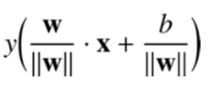Fig. 1

Well, to answer this question, consider the image in Fig. 2 below: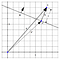Fig. 2

In Fig. 2 we see that the geometric margin of the point X, is the distance d between the point X and the point X’ on the hyperplane. To find the distance d, we can elicit the help of the vectors w and k on the hyperplane.

We can observe that since the vectors w and k both point in the same direction, they both have the same unit vector. Hence, the unit vector of w: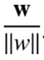Fig. 3

Can be used to express the vector k as: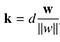Fig. 4

NOTE: A unit vector is a vector with a length of 1 unit. Hence to obtain the vector k, we have to multiply the unit vector d times.

Also, when you observe Fig. 2 again, you will observe that:

the vector X’ + the vector k = the vector X

therefore:

X’ = X-k

But we expressed k in Fig. 4 so we can substitute that expression here to get: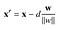Fig. 5

Now we know that since X’ is on the hyperplane, it satisfies the equation of the hyperplane, therefore w.X’ + b = 0.

But we have defined X’ in Fig. 5 and we can substitute its definition into the equation of the hyperplane to get: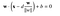Fig. 6

We can further perform an algebraic expansion of the equation in Fig. 6 to get the following:

Now, as we saw before, we can multiply by y to ensure that we select a hyperplane that correctly classifies the data, and gives the geometric margin formula:

In the next post, we will be talking about optimisation problems.

## More from David Sasu

https://sasudavid.github.io/dsasu/